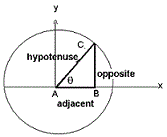Trigonometry Calculator

The trigonometric functions have been defined using the unit circle.

 Sin Cos Tan Cot Sec Cosec
 Arc Sin Arc Cos Arc Tan Arc Cot Arc Sec Arc Cosec

Degrees

 Sin Cos Tan Cot Sec Cosec
 Arc Sin Arc Cos Arc Tan Arc Cot Arc Sec Arc Cosec

The sin(θ) is the vertical component , the cos(θ) is the horizontal coordinate of the arc endpoint.

where

Sin(q) = Opposite / Hypotenuse